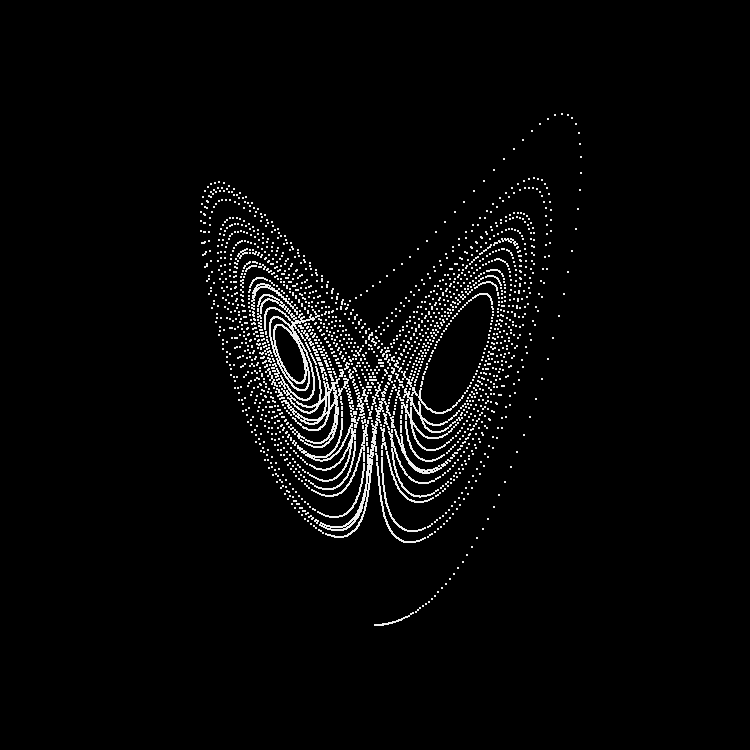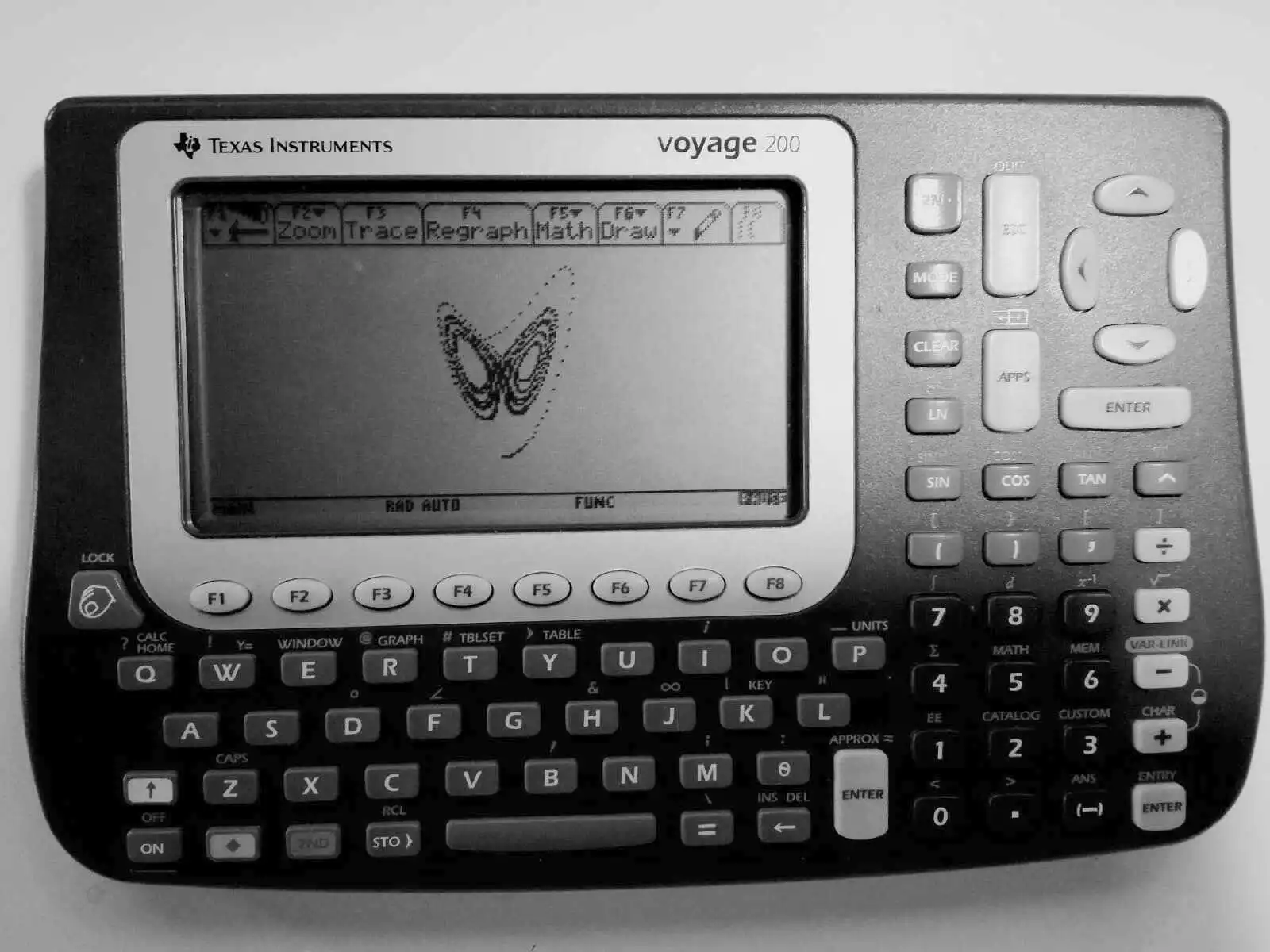# Lorenz Attractor

The first ever attractor of its kind was discovered by Edward Norton Lorenz in 1963 during research about weather behaviour and its predictability1. For a historical introduction to this attractor and chaos theory in general, I can recommend Chaos by James Gleick2. A well researched article by Quanta Magazine gives more insight to the daily work of Lorenz and his colleagues Ellen Fetter and Margaret Hamilton3.

## Renders

Differential system:

$\dot{x} = \alpha\, (y - x)$ $\dot{y} = x\, (\beta - z) - y$ $\dot{z} = x\, y - \gamma\, z$

Constants:

$\alpha = 10$ $\beta = 28$ $\gamma = 8/3$## Some Nostalgia

With just a few lines of code you can plot the attractor on BASIC-able devices. Here, I have tried it on a TI Voyage 200, a model from 2002 with just 188kB RAM and a 128p display:The program is in 68k TI-Basic4, which is a modified version by Texas Instruments and it looks like this:

lorenz()
Prgm
ClrDraw

.1 → x
.1 → y
.1 → z

10 → a
28 → b
8/3 → c

For i,0,1000
a*(y-x) → dx
x*(b-z)-y → dy
x*y-c*z → dz

x+dx/100 → x
y+dy/100 → y
z+dz/100 → z

PtOn x,z
EndFor

Pause
DispHome
EndPrgm


If you try this on the same model, the window settings were:

xmin = -82
xmax = 82
xscl = 1
ymin = -10
ymax = 60
yscl = 1


1. E.N. Lorenz, 1963. "Deterministic nonperiodic flow". J. Atmos. Sci. 20(2). doi:10.1175/1520-0469(1963)020<0130:DNF>2.0.CO;2

2. "Chaos: Making a New Science" by James Gleick (1988) on Goodreads

3. "The Hidden Heroines of Chaos" published in 2019 by Quanta Magazine

4. A great resource on the language is this forum/wiki

🕸 💍